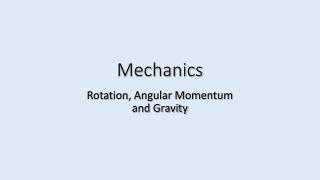# Mechanics - PowerPoint PPT PresentationDownload PresentationMechanics

MechanicsDownload Presentation## Mechanics

- - - - - - - - - - - - - - - - - - - - - - - - - - - E N D - - - - - - - - - - - - - - - - - - - - - - - - - - -
##### Presentation Transcript

1. Mechanics Rotation, Angular Momentum and Gravity

2. CH 9: Circular Motion

3. Rotational motion deals with an object moving along a curve. The analysis of the motion of an object as it moves along a curve is very similar to what was done for a straight line. We will essentially be looking at motion in terms of polar coordinates. B R Rotational motion is not only used for a circle. q B A A B Linear Motion Rotational Motion We are going to describe the motion in terms of angular position, angular velocity and angular acceleration. Angular position What are we describing when we use the term angular position? The location along a curve relative to a pre-defined zero angular position. How do we relate the distance along the curve (linear distance) to the angular position? s – Distance along curve [m] R – Radius of curvature [m] q – Angular position [radians] The angular position must be in radians. Do not use degrees! Circumference of a circle. This is valid when q = 2p.

4. If we know the initial and final angular positions we can determine the angular displacement. • – Final angular position [radians] • q0 – Initial angular position [radians] Angular Velocity The angular position can change in time. The rate of change of the angular position is called angular velocity. Angular velocity is the velocity along the curved path. – Average angular velocity [rad/s] w – Instantaneous angular velocity [rad/s] – Average angular acceleration [rad/s2] a – Instantaneous angular acceleration [rad/s2] Angular Acceleration The angular velocity can change in time. The rate of change of the angular velocity is called angular acceleration. Angular acceleration is the acceleration along the curved path.

5. For constant angular acceleration: These expressions are nearly identical to the constant acceleration equations for linear motion! We can also relate angular velocity and angular acceleration to linear velocity and linear acceleration. Relationship between tangential (linear) velocity and angular velocity. Relationship between tangential (linear) acceleration and angular acceleration. Centripetal acceleration along radial direction. Total linear acceleration.

6. The linear speed is the tangential speed at a point on the curve. The angular speed is the speed around the curve. s1 If we change the angular position of an object by q, how far has the object moved along each of the specified paths? s2 ¼ R q R Notice that the distance traveled for path s1 is greater than the distance traveled for path s2. Is the rate at which the angular position changes the same for both paths (assuming zero angular acceleration)? Yes, R sweeps out the same angular distance in the same time for both paths! If the distance traveled along the two paths is different, what does this say about the tangential velocity for each case? The tangential velocity will be larger for path s1 than for path s2! This is because a larger distance must be traveled along s1 in the same time interval. The angular displacement does not depend on the distance from the center of the curve. The path length does depend on the distance from the center of the curve. The angular velocity does not depend on the distance from the center of the curve. The tangential velocity does depend on the distance from the center of the curve. The angular acceleration does not depend on the distance from the center of the curve. The tangential acceleration does depend on the distance from the center of the curve.

7. Example: A person enters a stationary car at the bottom of a Ferris wheel. The ride is turned on and the car is given a constant angular acceleration of 0.5 rad/s2. The car is 20 m from the center of the Ferris wheel. • What is the angular speed of the car after 20 s? • What angular distance has the car traveled in this time? • What is the tangential speed of the car after 20 s? • What is the tangential speed of a light bulb on the arm of the Ferris wheel if it is located at a point ¼ the distance the car is from the center? 0 0 0 a) b) c) d)